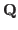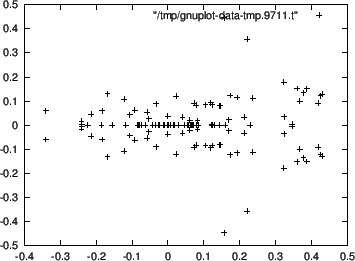### A Course on Solving Algebraic Equations

Risa/Asir  is a general computer algebra system which can be used for Gröbner basis computations for zero dimensional ideal withcoefficients. However, it is not good at graphical presentations and numerical methods. We integrated Risa/Asir, ox_phc (based on PHC pack by Verschelde  for the polyhedral homotopy method) and ox_gnuplot (GNUPLOT) servers to teach a course on solving algebraic equations. This course was presented with the text book , which discusses on the Gröbner basis method and the polyhedral homotopy method to solve systems of algebraic equations. We taught the course with a unified environment controlled by the Asir user language, which is similar to C. The following is an Asir session to solve algebraic equations by calling the PHC pack (Figure 2 is the output of ):

 phc(katsura(7));
The detailed output is in the file tmp.output.*
The answer is in the variable Phc.
0
 B=map(first,Phc)$ gnuplot_plotDots([],0)$
 gnuplot_plotDots(B,0)\$Nobuki Takayama 2017-03-30